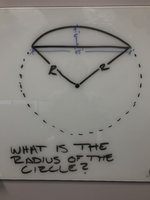# Finding the radius of a circle... impossible?

#### Bailey232008

##### New member
I’ve been working on this for too long. I almost posted this in the Odds and Ends section because I think it’s going to entail more than just geometry. Give this one a shot. Could be a fun challenge for you vets out there if it’s even possible. If you want the back story behind how I came up with it let me know.
The only information provided is what you see in the picture. Solve by any means necessary...#### MarkFL

##### Super Moderator
Staff member
By Heron, the area $$A$$ of the triangle is:

$$\displaystyle A=22.5\sqrt{(R+22.5)(R-22.5)}$$

We also know:

$$\displaystyle A=22.5(R-5)$$

This implies:

$$\displaystyle \sqrt{(R+22.5)(R-22.5)}=R-5$$

Solving this, we find:

$$\displaystyle R=53.125$$

•topsquark

#### pka

##### Elite Member
I’ve been working on this for too long. I almost posted this in the Odds and Ends section because I think it’s going to entail more than just geometry. Give this one a shot. Could be a fun challenge for you vets out there if it’s even possible. If you want the back story behind how I came up with it let me know.
The only information provided is what you see in the picture. Solve by any means necessary...View attachment 20083
On it we see $$R=\dfrac{c^2}{8h}+\dfrac{h}{2}$$
From the above diagram $$c=45'~\&~h=5'$$.

•topsquark

#### Jomo

##### Elite Member
Draw a line from where it says 45 to the center of the circle. This gives you a right triangle. What are the lengths of the three sides of this right triangle. Now ask Pythagorous for help.

•topsquark

#### Bailey232008

##### New member
Draw a line from where it says 45 to the center of the circle. This gives you a right triangle. What are the lengths of the three sides of this right triangle. Now ask Pythagorous for help.
You lack the interior angle, so this method doesn’t work

#### Bailey232008

##### New member
Mark and Pka,

great stuff, appreciate the help

#### Dr.Peterson

##### Elite Member
You lack the interior angle, so this method doesn’t work
You misunderstood Jomo's suggestion, which doesn't use any angles. In fact, this is how the formula pka gave is derived!

The sides of the triangle are r-h, c/2, and r (the hypotenuse), and solving the Pythagorean theorem for r yields the formula.

•topsquark

#### Jomo

##### Elite Member
You lack the interior angle, so this method doesn’t work
As already mentioned Pythagorous' theorem does not use angles. you have the 3 sides of the right triangle in terms of R. What seems to be the problem?

#### jonah2.0

##### Junior Member
Beer goggles hallucination follows.

Looked at the picture and saw 45° instead of 45'. Ended up thinking R as roughly 65.69.

#### Jomo

##### Elite Member
You lack the interior angle, so this method doesn’t work
Why couldn't you use the 45o angle that you thought was there?

#### yoscar04

##### Junior Member
Following Jomo's suggestion using straightforward Pythagoras theorem, you should get R=53.125.